Definitions of Square Dance Calls and Concepts

Index -->  Plus  |  A1  |  A2  |  C1  |  C2  |  C3A  |  C3B  |  C4  |  NOL  |
Definitions (Text Only) -->  Plus  |  A1  |  A2  |  C1  |  C2  |  C3A  |  C3B  |  C4  |  NOL  |
 Find call:

fraction Cast & Relay -- [C3B]
(author unknown)

\$BJ?9T\$J(B Wave \$B\$^\$?\$O(B Eight Chain Thru \$B\$+\$i(B.

1. \$BM?\$(\$i\$l\$?(B fraction \$B\$@\$1(B Arm Turn \$B\$r\$7(B;
2. Centers \$B\$,(B Cast Off 3/4 \$B\$r\$9\$k4V\$K(B, Ends \$B\$O(B 1/2 Circulate \$B\$r\$7(B;
3. Centers \$B\$,M?\$(\$i\$l\$?(B fraction \$B\$@\$1(B turn the Star \$B\$r\$9\$k4V\$K(B, \$BB>\$N?M\$O(B Trade \$B\$r\$7(B;
4. \$B9g\$C\$??M(B (Center Wave of 4) \$B\$,(B Cast Off 3/4 \$B\$r\$9\$k4V\$K(B, \$BB>\$N?M\$O(B move up (Phantom Hourglass Circulate) \$B\$r\$7\$^\$9(B.

\$BJ?9T\$J(B Wave \$B\$G=*\$o\$j\$^\$9(B.

fraction \$B\$r>JN,\$9\$k\$3\$H\$O\$G\$-\$^\$;\$s(B.

1/4 Cast & Relay: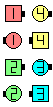1/4 Cast & Relay\$B\$NA0(B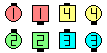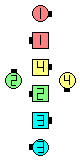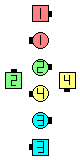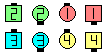Arm Turn 1/4\$B\$N8e(B Centers Cast Off 3/4 as Ends 1/2 Circulate\$B\$N8e(B Centers Star 1/4 as Outsides Trade\$B\$N8e(B Those who meet Cast Off 3/4as Others move up\$B\$N8e(B (\$B=*\$o\$j(B)

\$BCm
• Cast & Relay \$B\$O(B Relay The Top [C1] \$B\$N(B, \$B;O\$a\$N(B Arm Turn \$B\$H(B Star \$B\$N2s\$kEY9g\$\$\$rM?\$(\$i\$l\$?(B fraction \$B\$K\$7\$?\$b\$N\$G\$9(B.
• 1/4 Cast & Relay \$B\$KCm0U\$7\$F\$/\$@\$5\$\$(B. \$B\$J\$<\$J\$i(B, 2 \$B\$D\$N(B 1/4 turn \$B\$H(B 2 \$B\$D\$N(B 3/4 turn \$B\$,\$"\$k\$+\$i\$G\$9(B.
• 1/2 Cast & Relay \$B\$O(B Relay The Top, Star 1/2 \$B\$HF1\$8\$G\$9(B.
• \$B1~MQ(B: \$B1~MQ(B: Star 1/4; Star 1/2; Star 3/4; Don't Turn The Star.

fraction Cast & Relay But anything [C3BV]: fraction Cast & Relay \$B\$r\$7\$^\$9\$,(B, \$B:G8e\$N(B Centers Cast Off 3/4 \$B\$r(B Centers \$B\$,(B anything \$B\$N%3!<%k\$G(B replace \$B\$7\$^\$9(B.

{i - j - k - l} Relay The Top [C4]: \$BJ?9T\$J(B Wave \$B\$^\$?\$O(B Eight Chain Thru \$B\$+\$i(B. Relay The Top \$B\$N:G=i\$N(B 1/2 Turn \$B\$r!!(Bi/4 Turn \$B\$GCV\$-49\$((B; Centers Cast Off 3/4 \$B\$r!!(Bj/4 Turn \$B\$K49\$(\$F(B; turn the Star k/4 \$B\$r\$7\$F(B; \$B:G8e\$N(B Centers Cast Off 3/4 \$B\$r!!(Bl/4 Turn \$B\$KCV\$-49\$(\$^\$9(B. \$BJ?9T\$J(B wave \$B\$^\$?\$O(B 2 \$B\$D\$N(B Facing Diamond \$B\$G=*\$o\$j\$^\$9(B. \$B\$3\$l\$O(B Outsides Trade \$B\$r=|\$1\$P(B {i-j-k-l} 1/4 The Deucey [C4] \$B\$HF1\$8\$G\$9(B.Choreography for fraction Cast & RelayComments? Questions? Suggestions?

https://www.ceder.net/def/castandrelay.php?language=japan&level=C3B
14-August-2020 21:50:27